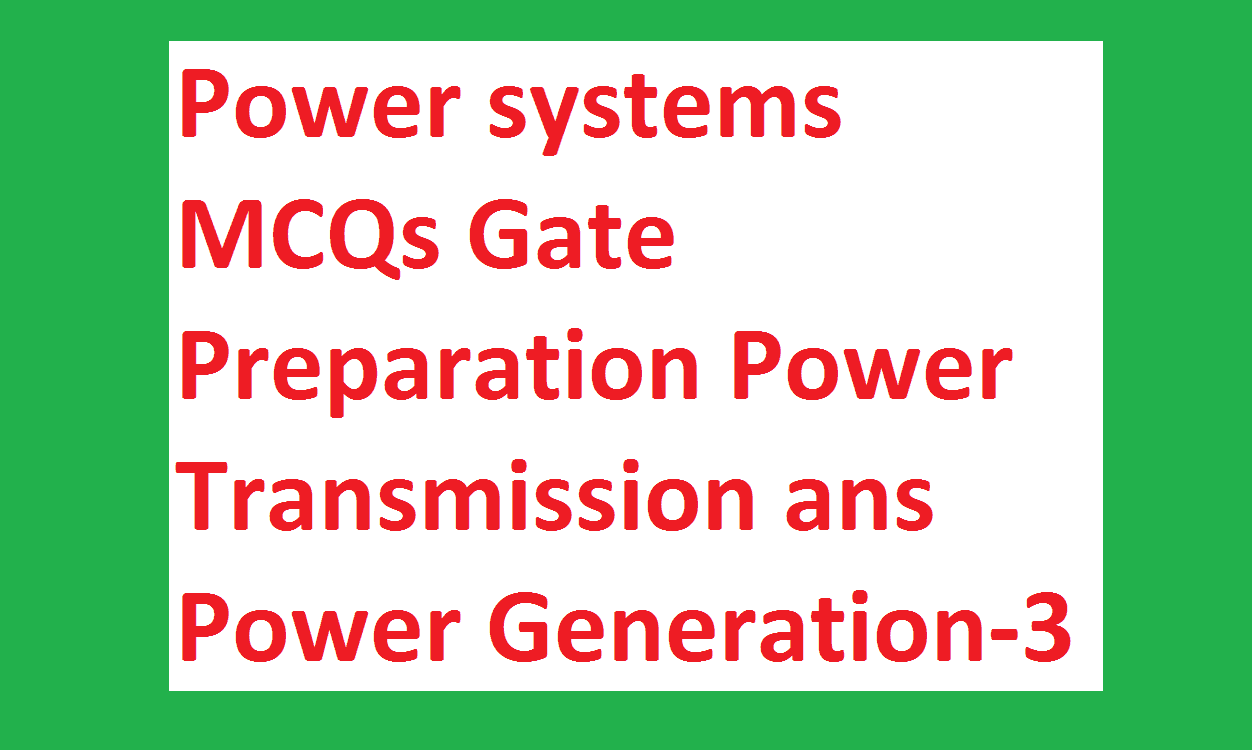# Power systems MCQs Gate Preparation Power Transmission Generation-3:

51.A voltage of 1000 kV is applied to an overhead line with its receiving end open. If the surge impedance of the line is 500 ohms, then the total surge power in the line is
a)2000MW b)500MW
c)2MW d)0.5MW

52.Shunt compensation in an EHV line is used
a) improve stability
b) reduce fault level
c)improve the voltage
d)substitute for synchronous phase modifier
Answer: substitute for synchronous phase modifier.

53.An industrial consumer has a daily load pattern of 2000kW,0.8 lag for 12 Hrs. and 1000kW UPF for 12 Hrs. the load factor is
a)10.5 b)0.75
c)0.6 d)2.0

54.The single phase line of 230 V and 3-phase line of 400 V which feed different domestic circuits and industrial loads represent
a) primary distribution
b) secondary distribution
c)secondary transmission
d)none of these

55.The transmission line feeding power on either side of the main transmission line is called
a) secondary distribution
b) secondary transmission
c)primary transmission
d)primary distribution

56.Series capacitive compensation on EHV transmission lines is used to
b) improve the stability of the system
c)reduce the voltage profile
d)improve the protection of the line
Answer: improve the protection of the line

57.At a particular unbalanced node, the real powers specified are
Leaving the node:20 MW,25 MW,
Entering the node: 60 MW, 30 MW.
The balance power will be
a)30 MW leaving the node
b)45 MW leaving the node
c)45 MW entering the node
d)22.5MW entering the node and 22.5 MW leaving the node
Answer: 45 MW leaving the node

58.When a deck source is switched is purely inductive, the current response is
a) an exponentially rising is
b) an exponentially decaying curve
c)a straight line passing through the origin
d)a straight line off-set from the origin
Answer: a straight line passing through the origin

59.If transformer frequency is changed from 50 Hz, to 60 Hz the ratio of eddy current loss 60 Hz, to 50 Hz at constant voltage will be
a)5/6 b)25/36
c)6/5 d)1.060.In load flow analysis, the load connected at a bus is represented as
a) constant current drawn from the bus
b) constant impedance connected at the bus
c)voltage and frequency dependent source at the bus
d)constant real and reactive drawn from the bus
Answer: constant real and reactive drawn from the bus

## VOLTAGE CONTROL AND POWER FACTOR CORRECTION

What is Power factor

61.In a synchronous machine, in case the axis of field flux is in line with the armature flux, the machine is working
a) as synchronous mother
b) as the synchronous generator
c)as floating machine
d)the machine will not work

62.An ideal voltage source is connected across a variable resistance. The variation of current as a function of resistance is given by
a) a straight line passing through the origin
b) a rectangular hyperbola
c)a parabola
d)all of the above

63.Pure inductive circuit takes power (reactive) from the arc. line when
a) both applied voltage and current rise
b) both applied voltage and current decrease
c)applied voltage decreases but current increases
d)none of these
Answer: both applied voltage and current decrease

64.An alternator has a phase sequence of RYB for its phase voltage. In case of field current is reversed, the phase sequence will become
a) RBY b) RYB
c)YRB d) none of these

Learn More:   Copper Loss Calculation Calculator for Single Phase & Three Phase Transformer

65.A 10 kVA, 400 V/200 V single phase transformer with 10% impedance draws a steady short circuit line current of
a)50 A b)150 A
c)250 A d)350 A

66.The percentage resistance and percentage reactance of a 10 kVA, 400 V/200 V, 3-phase transformer are 2% and 10% respectively. If the constant losses in the machine are 1%, the maximum possible percentage efficiency of the transformer is
a)98.32 b)97.25
c)96.85 d)96.12

67.With the help of a reactive compensator, it is possible to have
a) constant voltage operation only
b) unity pp. operation only
c)both constant voltage and unity pp.
d)either constant voltage or unity pp.
Answer: either constant voltage or unity pp.

68.For a long uncompensated line the limit to the line loading is governed by
a) thermal limit b) voltage drop
c)stability limit d) corona loss

69.A shunt reactor at 100 Mar is operated at 98% of its rated voltage and at 96% of its rated frequency. The reactive power absorbed by the reactor is
a)98 Mar b)104.02 Mar
c)96.04 Mar d)100.04 Mar

70.A 100 kVA transformer has 4% impedance and 50 kVA transformer has 3% impedance when they are operated in parallel, which transformer will reach full load first
a)3% b)4%
c)data is insufficient d) none of these

71.The combined frequency regulation of machines in area of capacity 150 MW and operating at a normal frequency of 60 kHz is 0.1 up on its own base capacity. The regulation in Hz/MW will be
a)0.1/1500 b)60/1500
c)6/1500 d)60/150

72.Load frequency control is achieved by properly matching the individual machine’s
a) reactive powers
b) generated voltages
c)turbine inputs
d)turbine and generator ratings

73.In a power station, the cost of generation of power reduces most effectively when
a) diversity factor alone increase
b) both diversity factor and load factor increases
d)both diversity factor and load factor decreases

74.The additional cost per hour to increase the output of plant 1 by 1 MW is
a)200 Rs/MWh b)151 Rs/MWh
c)160 Rs/MWh d)180 Rs/MWh